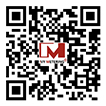Добро пожаловать в Ivy Metering!# What is Four Quadrant Metrology?

Author: YiWei66 Time: 09. 21, 2023 Classify: 2023

According to the provisions of DL/T645-2007 of "Multifunctional Energy Meter Communication Protocol", we define the horizontal axis of a plane coordinate system as active power, and the vertical axis as reactive power. The two axes divide a plane into four areas , the one in the upper right corner is quadrant I, and the clockwise order is quadrant II, quadrant III and quadrant IV; the reactive power in quadrant I and quadrant II is defined as forward reactive power, and the reactive power in quadrant III and quadrant IV is defined as reverse reactive power;

and has a phase angle Φ with respect to the voltage phasor U. The angle Φ in the counterclockwise direction is positive. A schematic diagram of four quadrants is shown:P—active energy; Q—reactive energy; QL—inductive reactive energy; QC—capacitive reactive energy; S—apparent power

Forward active power: P=UICOSΦ, where the range of Φ is within ±90°.

Reverse active power: P=UICOSΦ, where Φ ranges from 90° to 270°

Forward reactive power: P= UISINΦ, where the range of Φ is within 0~180°

Reverse reactive power: P= UISINΦ, where the range of Φ is within 180~360°

Since the voltage leads the current within 90° in the first quadrant, and in the pure inductance circuit, the voltage leads the current by 90°, so when the input (forward) active power is active, the reactive power generated in the first quadrant is called inductive Reactive power; since the voltage lags the current within 90° in the IV quadrant, and in the pure capacitive circuit, the voltage lags the current by 90°, so when the active power is input, the reactive power generated in the IV quadrant is called capacitive No use. Similarly, when outputting (reverse) active power, the third quadrants are inductive reactive power and capacitive reactive power respectively.

Power is expressed in four quadrants:

1) Forward active power: that is, the input active power, which is the power sent by the grid to the user, and is the power consumed by the user;

2) Reverse active power: that is, the output active power, which is the user's power transmission to the grid and the power generated by the user;

3) Forward reactive power: that is, the input reactive power, which is the reactive power sent by the grid to the user and the reactive power used by the user;

4) Reverse reactive power: that is, the output reactive power, which is the reactive power sent by the user to the grid, and the reactive power sent by the user;

The meaning of reactive power in four quadrants:

1) Quadrant Ⅰ reactive power: input active power, input reactive power, the user is an inductive (current lagging voltage) load;

2) Quadrant II reactive power: output active power, input reactive power, the user load is equivalent to an under-excited generator;

3) Quadrant III reactive power: output active power, output reactive power, the user load is equivalent to an over-excitation generator;

4) Quadrant IV reactive power: input active power, output reactive power, the user is a capacitive (current ahead of voltage) load;

The meaning of capacitive reactive power and perceptual reactive power:

1) Quadrant I: consumption of active power and consumption of inductive reactive power.

2) Quadrant II: output active power and output capacitive reactive power.

3) Ⅲ quadrant: output active power, output inductive reactive power.

4) Quadrant IV: consumption of active power and consumption of capacitive reactive power.